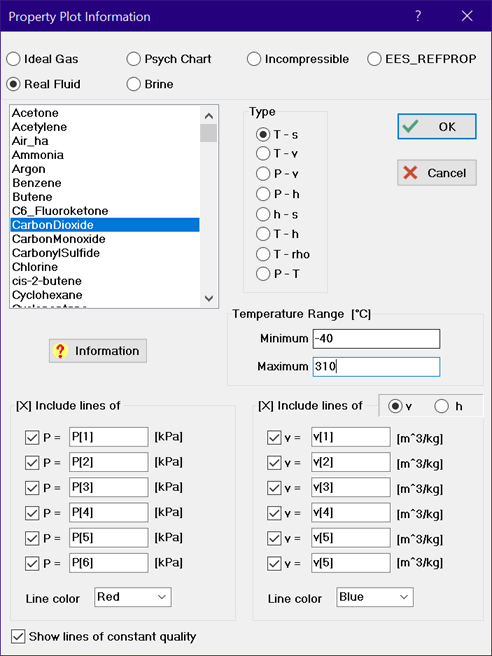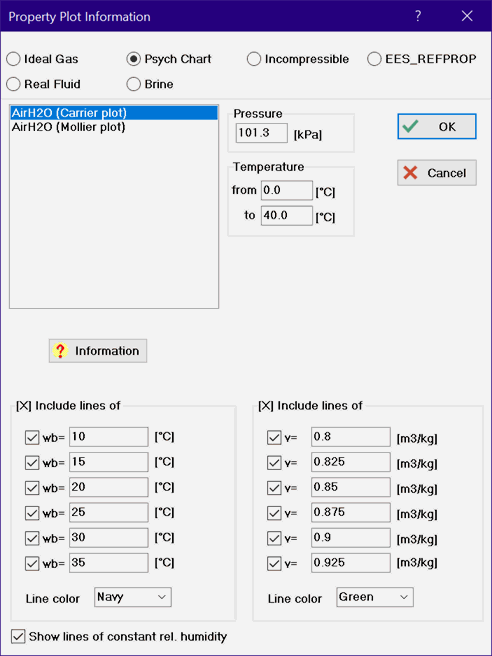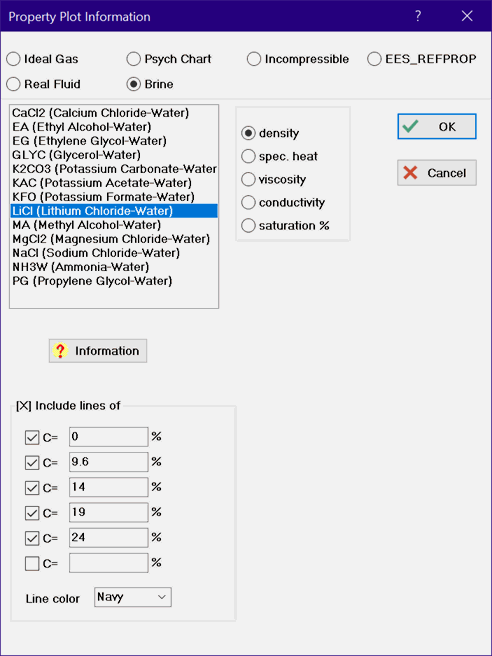Contents - Index

Property Plot

The Property Plot command creates a new plot window with thermodynamic property data for a selected substance.  Once created, additional property data or thermodynamic cycle state points can be superimposed on the plot using the Plot_Overlay_Plot command.Select the substance type from the radio buttons at the top of the dialog.  Then select the substance from the list at the left.  Detailed information relating to the substance model can be obtained by clicking on the Fluid Info button below the list.

For Ideal Gas and Real Fluid substances, seven buttons are shown that allow a specification of Temperature-entropy (T-s), Temperature-volume (T-v), Pressure-volume (P-v),  Pressure-enthalpy (P-h),  Enthalpy-pressure (h-s),Temperature-enthalpy (T-h) or Temperature-density (T-rho) coordinate systems.  The T-h plot option is available only for substances that are represented by the high accuracy fundamental equation of state.  These substances are identified in the Fluid Property Information window.  Several sets of controls will appear in which 6 values of additional properties, such as isobars, isotherms, and/or isentropes can be specified.  Suggested values of these properties may be provided in the edit boxes.  To display lines of constant properties, click the check box so that an X appears in the box and then enter or modify the existing value for the property.   EES variables that have defined values can be used in place of numerical values (starting with version 10.653) as demonstrated in the dialog for CarbonDioxide having a specified pressure line of P..  The color of these additional property lines can be selected from the drop-down controls below the 6 values.

Fluids modeled with a high-accuracy equation of state allow the reference state for enthalpy and entropy to be selected, as shown in the figure on the left.  The options are DFT, NBP, ASH, and IIR.  These alternatives are defined in the discussion of the \$Reference directive.  When plotting T-S, T-V or T-H plots, a default maximum temperature is chosen that is often less that the maximum temperature for which the property correlation is valid.  The maximum temperature used in preparing the property plot can be adjusted in these cases using either the edit box or the slider control in the Max. Temperature box as shown in the property plot setup for CarbonDioxide on the left.

Selection of the Psych Chart button will result in a psychrometric plot for substance AIRH2O in either the Carrier or Mollier format as shown above in the figure in the center.  AIRH20 allows lines of constant relative humidity, web-bulb temperature and specific volume to be placed on a psychrometric chart.

Selection of the Brines button will provide the option of plotting density, specific heat, viscosity or thermal conductivity as a function of temperature for up to six specified mass concentrations of the brine in water.  See the above figure on the right.

Calculated property data can be superimposed on a property plot using the Overlay Plot command.  To construct plots of this nature, it is most convenient to place the properties that are to be plotted in arrays using square brackets to enclose the array index, e.g., T.  In that way, the property values will be displayed in the Arrays window after calculations are completed and they can then be directly plotted.   Also, the plot characteristics and axis scales can be modified in the usual manner with the Modify Axes and Modify Plot commands.